Chemistry

# Chapter 7

ChemistryChapter 7

1.

The protons in the nucleus do not change during normal chemical reactions. Only the outer electrons move. Positive charges form when electrons are lost.

3.

P, I, Cl, and O would form anions because they are nonmetals. Mg, In, Cs, Pb, and Co would form cations because they are metals.

5.

(a) P3–; (b) Mg2+; (c) Al3+; (d) O2–; (e) Cl; (f) Cs+

7.

(a) [Ar]4s23d104p6; (b) [Kr]4d105s25p6 (c) 1s2 (d) [Kr]4d10; (e) [He]2s22p6; (f) [Ar]3d10; (g) 1s2 (h) [He]2s22p6 (i) [Kr]4d105s2 (j) [Ar]3d7 (k) [Ar]3d6, (l) [Ar]3d104s2

9.

(a) 1s22s22p63s23p1; Al3+: 1s22s22p6; (b) 1s22s22p63s23p63d104s24p5; 1s22s22p63s23p63d104s24p6; (c) 1s22s22p63s23p63d104s24p65s2; Sr2+: 1s22s22p63s23p63d104s24p6; (d) 1s22s1; Li+: 1s2; (e) 1s22s22p63s23p63d104s24p3; 1s22s22p63s23p63d104s24p6; (f) 1s22s22p63s23p4; 1s22s22p63s23p6

11.

NaCl consists of discrete ions arranged in a crystal lattice, not covalently bonded molecules.

13.

ionic: (b), (d), (e), (g), and (i); covalent: (a), (c), (f), (h), (j), and (k)

15.

(a) Cl; (b) O; (c) O; (d) S; (e) N; (f) P; (g) N

17.

(a) H, C, N, O, F; (b) H, I, Br, Cl, F; (c) H, P, S, O, F; (d) Na, Al, H, P, O; (e) Ba, H, As, N, O

19.

N, O, F, and Cl

21.

(a) HF; (b) CO; (c) OH; (d) PCl; (e) NH; (f) PO; (g) CN

23.

(a) eight electrons: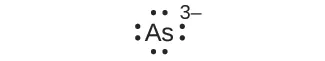;
(b) eight electrons: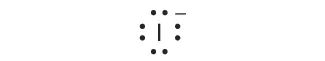;
(c) no electrons
Be2+;
(d) eight electrons:;
(e) no electrons
Ga3+;
(f) no electrons
Li+;
(g) eight electrons: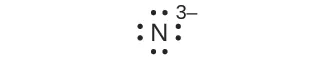25.

(a)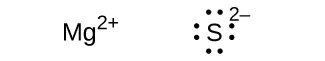;
(b)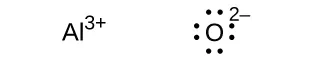;
(c)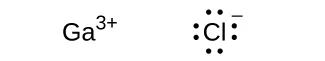;
(d)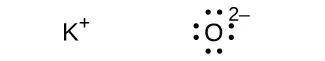;
(e)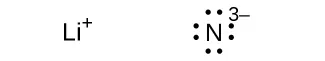;
(f)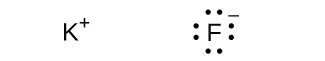27.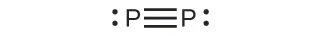29.

(a)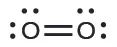In this case, the Lewis structure is inadequate to depict the fact that experimental studies have shown two unpaired electrons in each oxygen molecule.
(b);
(c)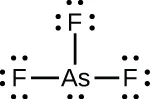;
(d)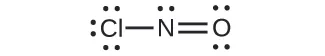;
(e);
(f)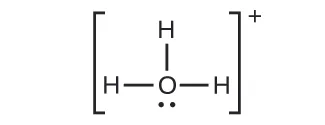;
(g)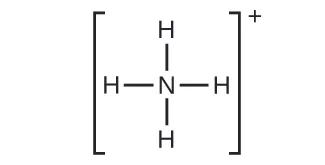;
(h)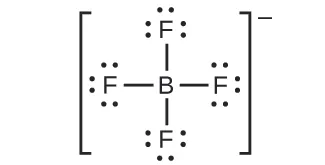;
(i);
(j)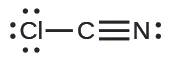;
(k)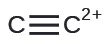31.

(a) SeF6: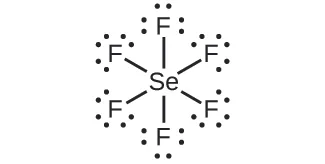;
(b) XeF4: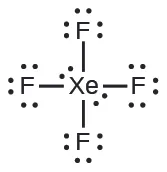;
(c) $SeCl3+:SeCl3+:$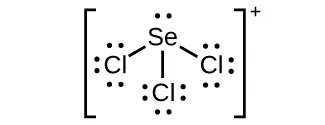;
(d) Cl2BBCl2:33.

Two valence electrons per Pb atom are transferred to Cl atoms; the resulting Pb2+ ion has a 6s2 valence shell configuration. Two of the valence electrons in the HCl molecule are shared, and the other six are located on the Cl atom as lone pairs of electrons.

35.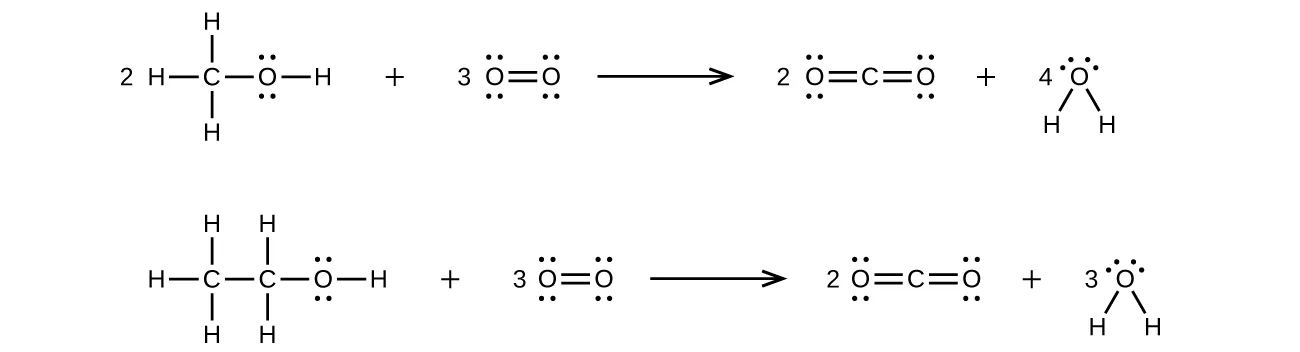37.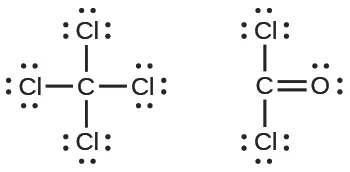39.

(a)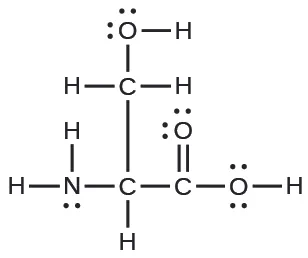;
(b);
(c)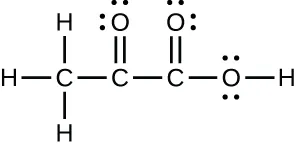;
(d)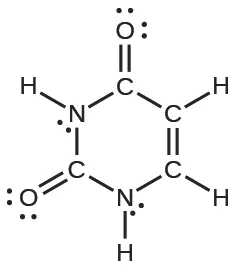;
(e)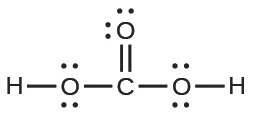41.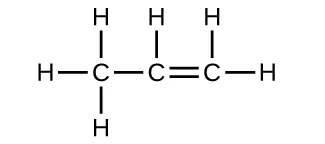43.

Each bond includes a sharing of electrons between atoms. Two electrons are shared in a single bond; four electrons are shared in a double bond; and six electrons are shared in a triple bond.

45.

(a)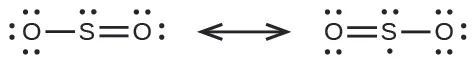;
(b)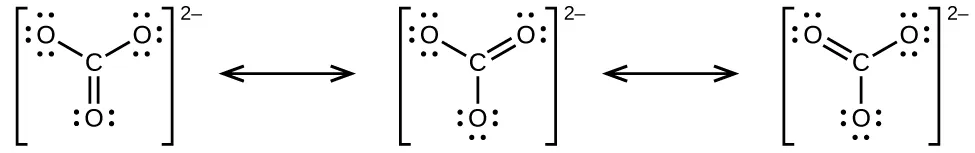;
(c)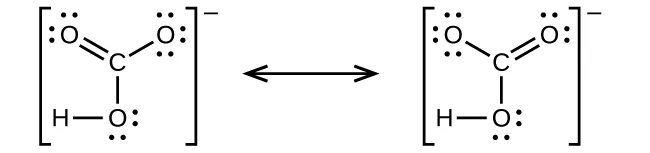;
(d);
(e)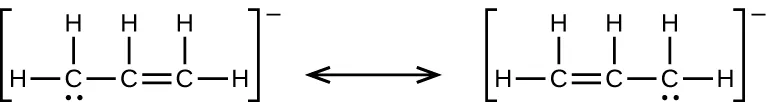47.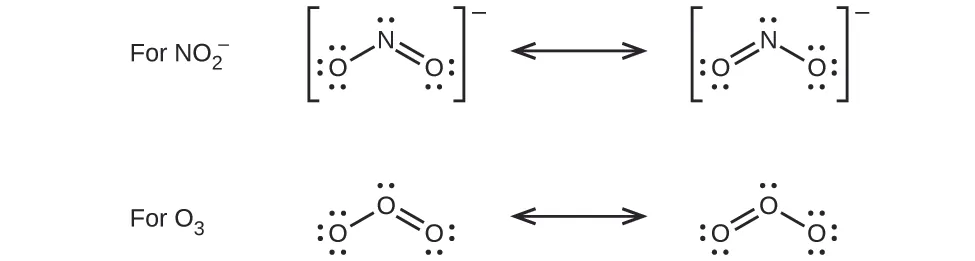49.

(a)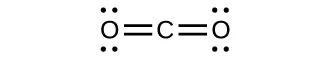(b)CO has the strongest carbon-oxygen bond because there is a triple bond joining C and O. CO2 has double bonds.

51.

(a) H: 0, Cl: 0; (b) C: 0, F: 0; (c) P: 0, Cl 0; (d) P: 0, F: 0

53.

Cl in Cl2: 0; Cl in BeCl2: 0; Cl in ClF5: 0

55.

(a);
(b);
(c);
(d)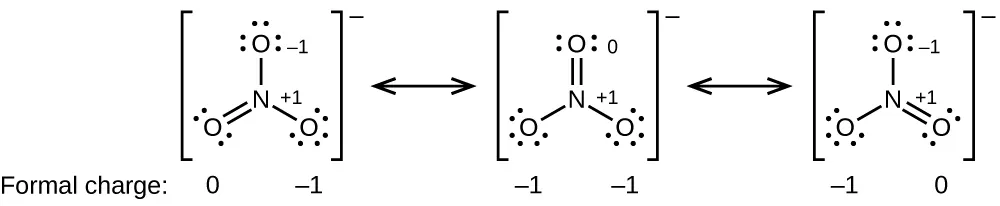57.

HOCl

59.

The structure that gives zero formal charges is consistent with the actual structure: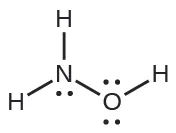61.

NF3;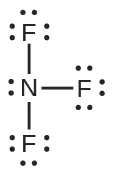63.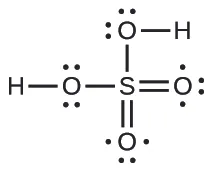65.

(a) −114 kJ;
(b) 30 kJ;
(c) −1055 kJ

67.

The greater bond energy is in the figure on the left. It is the more stable form.

69.

$HCl(g)⟶12H2(g)+12Cl2(g)ΔH1°=−ΔHf[HCl(g)]°12H2(g)⟶H(g)ΔH2°=ΔHf[H(g)]°12Cl2(g)⟶Cl(g)ΔH3°=ΔHf[Cl(g)]°¯HCl(g)⟶H(g)+Cl(g)ΔH298°=ΔH1°+ΔH2°+ΔH3°HCl(g)⟶12H2(g)+12Cl2(g)ΔH1°=−ΔHf[HCl(g)]°12H2(g)⟶H(g)ΔH2°=ΔHf[H(g)]°12Cl2(g)⟶Cl(g)ΔH3°=ΔHf[Cl(g)]°¯HCl(g)⟶H(g)+Cl(g)ΔH298°=ΔH1°+ΔH2°+ΔH3°$
$DHCl=ΔH298°=ΔHf[HCl(g)]°+ΔHf[H(g)]°+ΔHf[Cl(g)]°=−(−92.307kJ)+217.97kJ+121.3kJ=431.6kJDHCl=ΔH298°=ΔHf[HCl(g)]°+ΔHf[H(g)]°+ΔHf[Cl(g)]°=−(−92.307kJ)+217.97kJ+121.3kJ=431.6kJ$

71.

The S–F bond in SF4 is stronger.

73.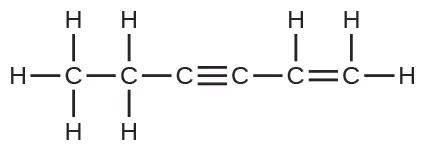The C–C single bonds are longest.

75.

(a) When two electrons are removed from the valence shell, the Ca radius loses the outermost energy level and reverts to the lower n = 3 level, which is much smaller in radius. (b) The +2 charge on calcium pulls the oxygen much closer compared with K, thereby increasing the lattice energy relative to a less charged ion. (c) Removal of the 4s electron in Ca requires more energy than removal of the 4s electron in K because of the stronger attraction of the nucleus and the extra energy required to break the pairing of the electrons. The second ionization energy for K requires that an electron be removed from a lower energy level, where the attraction is much stronger from the nucleus for the electron. In addition, energy is required to unpair two electrons in a full orbital. For Ca, the second ionization potential requires removing only a lone electron in the exposed outer energy level. (d) In Al, the removed electron is relatively unprotected and unpaired in a p orbital. The higher energy for Mg mainly reflects the unpairing of the 2s electron.

77.

(d)

79.

4008 kJ/mol; both ions in MgO have twice the charge of the ions in LiF; the bond length is very similar and both have the same structure; a quadrupling of the energy is expected based on the equation for lattice energy

81.

(a) Na2O; Na+ has a smaller radius than K+; (b) BaS; Ba has a larger charge than K; (c) BaS; Ba and S have larger charges; (d) BaS; S has a larger charge

83.

(e)

85.

The placement of the two sets of unpaired electrons in water forces the bonds to assume a tetrahedral arrangement, and the resulting HOH molecule is bent. The HBeH molecule (in which Be has only two electrons to bond with the two electrons from the hydrogens) must have the electron pairs as far from one another as possible and is therefore linear.

87.

Space must be provided for each pair of electrons whether they are in a bond or are present as lone pairs. Electron-pair geometry considers the placement of all electrons. Molecular structure considers only the bonding-pair geometry.

89.

As long as the polar bonds are compensated (for example. two identical atoms are found directly across the central atom from one another), the molecule can be nonpolar.

91.

(a) Both the electron geometry and the molecular structure are octahedral.
(b) Both the electron geometry and the molecular structure are trigonal bipyramid.
(c) Both the electron geometry and the molecular structure are linear.
(d) Both the electron geometry and the molecular structure are trigonal planar.

93.

(a) electron-pair geometry: octahedral, molecular structure: square pyramidal; (b) electron-pair geometry: tetrahedral, molecular structure: bent; (c) electron-pair geometry: octahedral, molecular structure: square planar; (d) electron-pair geometry: tetrahedral, molecular structure: trigonal pyramidal; (e) electron-pair geometry: trigonal bypyramidal, molecular structure: seesaw; (f) electron-pair geometry: tetrahedral, molecular structure: bent (109°)

95.

(a) electron-pair geometry: trigonal planar, molecular structure: bent (120°); (b) electron-pair geometry: linear, molecular structure: linear; (c) electron-pair geometry: trigonal planar, molecular structure: trigonal planar; (d) electron-pair geometry: tetrahedral, molecular structure: trigonal pyramidal; (e) electron-pair geometry: tetrahedral, molecular structure: tetrahedral; (f) electron-pair geometry: trigonal bipyramidal, molecular structure: seesaw; (g) electron-pair geometry: tetrahedral, molecular structure: trigonal pyramidal

97.

All of these molecules and ions contain polar bonds. Only ClF5, $ClO2−,ClO2−,$ PCl3, SeF4, and $PH2−PH2−$ have dipole moments.

99.

SeS2, CCl2F2, PCl3, and ClNO all have dipole moments.

101.

P

103.

nonpolar

105.

(a) tetrahedral; (b) trigonal pyramidal; (c) bent (109°); (d) trigonal planar; (e) bent (109°); (f) bent (109°); (g) CH3CCH tetrahedral, CH3CCH linear; (h) tetrahedral; (i) H2CCCH2 linear; H2CCCH2 trigonal planar

107.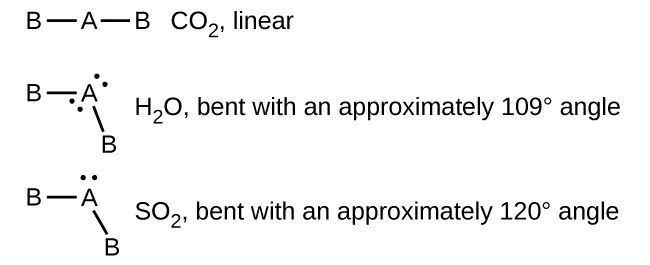109.

(a)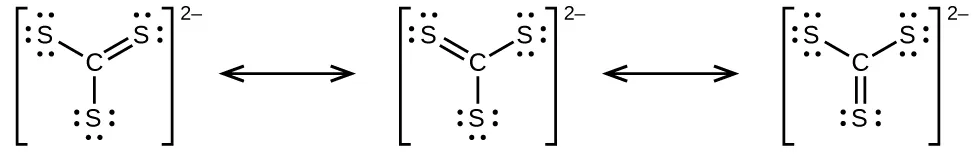;
(b)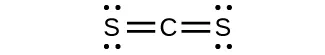;
(c)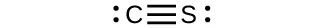;
(d) $CS32−CS32−$ includes three regions of electron density (all are bonds with no lone pairs); the shape is trigonal planar; CS2 has only two regions of electron density (all bonds with no lone pairs); the shape is linear

111.

The Lewis structure is made from three units, but the atoms must be rearranged: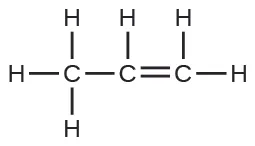113.

The molecular dipole points away from the hydrogen atoms.

115.

The structures are very similar. In the model mode, each electron group occupies the same amount of space, so the bond angle is shown as 109.5°. In the “real” mode, the lone pairs are larger, causing the hydrogens to be compressed. This leads to the smaller angle of 104.5°.

Order a print copy

As an Amazon Associate we earn from qualifying purchases.